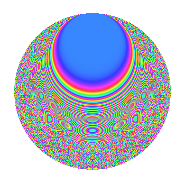# Properties

 Label 1175.4.aLevel 1175 Weight 4 Character orbit a Rep. character $$\chi_{1175}(1,\cdot)$$ Character field $$\Q$$ Dimension 219 Newform subspaces 12 Sturm bound 480 Trace bound 2

# Related objects

## Defining parameters

 Level: $$N$$ = $$1175 = 5^{2} \cdot 47$$ Weight: $$k$$ = $$4$$ Character orbit: $$[\chi]$$ = 1175.a (trivial) Character field: $$\Q$$ Newform subspaces: $$12$$ Sturm bound: $$480$$ Trace bound: $$2$$ Distinguishing $$T_p$$: $$2$$

## Dimensions

The following table gives the dimensions of various subspaces of $$M_{4}(\Gamma_0(1175))$$.

Total New Old
Modular forms 366 219 147
Cusp forms 354 219 135
Eisenstein series 12 0 12

The following table gives the dimensions of the cuspidal new subspaces with specified eigenvalues for the Atkin-Lehner operators and the Fricke involution.

$$5$$$$47$$FrickeDim.
$$+$$$$+$$$$+$$$$59$$
$$+$$$$-$$$$-$$$$44$$
$$-$$$$+$$$$-$$$$53$$
$$-$$$$-$$$$+$$$$63$$
Plus space$$+$$$$122$$
Minus space$$-$$$$97$$

## Trace form

 $$219q - 6q^{2} + 2q^{3} + 890q^{4} + 32q^{6} + 18q^{7} - 30q^{8} + 1959q^{9} + O(q^{10})$$ $$219q - 6q^{2} + 2q^{3} + 890q^{4} + 32q^{6} + 18q^{7} - 30q^{8} + 1959q^{9} + 70q^{11} - 7q^{12} - 40q^{13} + 205q^{14} + 3538q^{16} + 138q^{17} + 35q^{18} - 70q^{19} - 64q^{21} + 218q^{22} - 24q^{23} + 863q^{24} - 476q^{26} + 56q^{27} + 660q^{28} - 496q^{29} - 132q^{31} + 339q^{32} + 432q^{33} - 216q^{34} + 7618q^{36} + 566q^{37} - 820q^{38} + 460q^{39} + 226q^{41} + 463q^{42} + 402q^{43} - 1316q^{44} - 874q^{46} - 235q^{47} - 860q^{48} + 10855q^{49} - 222q^{51} - 60q^{52} + 454q^{53} + 1125q^{54} + 3244q^{56} + 764q^{57} + 520q^{58} + 162q^{59} + 2542q^{61} + 324q^{62} + 1628q^{63} + 14134q^{64} + 2068q^{66} + 658q^{67} + 2113q^{68} - 1532q^{69} + 522q^{71} - 1254q^{72} - 2462q^{73} + 3064q^{74} - 3750q^{76} + 1556q^{77} - 824q^{78} - 1498q^{79} + 20595q^{81} + 2126q^{82} + 1440q^{83} - 1199q^{84} + 2036q^{86} - 4408q^{87} + 2920q^{88} + 3398q^{89} + 1508q^{91} + 84q^{92} + 6484q^{93} + 376q^{94} - 1484q^{96} + 2738q^{97} - 2234q^{98} + 5542q^{99} + O(q^{100})$$

## Decomposition of $$S_{4}^{\mathrm{new}}(\Gamma_0(1175))$$ into newform subspaces

Label Dim. $$A$$ Field CM Traces A-L signs $q$-expansion
$$a_2$$ $$a_3$$ $$a_5$$ $$a_7$$ 5 47
1175.4.a.a $$3$$ $$69.327$$ 3.3.1101.1 None $$5$$ $$5$$ $$0$$ $$45$$ $$+$$ $$+$$ $$q+(2+\beta _{2})q^{2}+(1+\beta _{1}-\beta _{2})q^{3}+(2+\cdots)q^{4}+\cdots$$
1175.4.a.b $$8$$ $$69.327$$ $$\mathbb{Q}[x]/(x^{8} - \cdots)$$ None $$-3$$ $$-7$$ $$0$$ $$-39$$ $$+$$ $$-$$ $$q-\beta _{1}q^{2}+(-1-\beta _{5})q^{3}+(5+\beta _{2}-\beta _{3}+\cdots)q^{4}+\cdots$$
1175.4.a.c $$8$$ $$69.327$$ $$\mathbb{Q}[x]/(x^{8} - \cdots)$$ None $$3$$ $$10$$ $$0$$ $$15$$ $$+$$ $$-$$ $$q+\beta _{1}q^{2}+(2-\beta _{1}-\beta _{3})q^{3}+(1+\beta _{1}+\cdots)q^{4}+\cdots$$
1175.4.a.d $$10$$ $$69.327$$ $$\mathbb{Q}[x]/(x^{10} - \cdots)$$ None $$-7$$ $$-8$$ $$0$$ $$-9$$ $$+$$ $$-$$ $$q+(-1+\beta _{1})q^{2}+(-1+\beta _{6})q^{3}+(4+\cdots)q^{4}+\cdots$$
1175.4.a.e $$13$$ $$69.327$$ $$\mathbb{Q}[x]/(x^{13} - \cdots)$$ None $$3$$ $$10$$ $$0$$ $$19$$ $$+$$ $$+$$ $$q+\beta _{1}q^{2}+(1+\beta _{4})q^{3}+(4+\beta _{2})q^{4}+\cdots$$
1175.4.a.f $$15$$ $$69.327$$ $$\mathbb{Q}[x]/(x^{15} - \cdots)$$ None $$-7$$ $$-8$$ $$0$$ $$-13$$ $$+$$ $$+$$ $$q-\beta _{1}q^{2}+(-1-\beta _{4})q^{3}+(5+\beta _{2})q^{4}+\cdots$$
1175.4.a.g $$18$$ $$69.327$$ $$\mathbb{Q}[x]/(x^{18} - \cdots)$$ None $$-1$$ $$-7$$ $$0$$ $$-6$$ $$+$$ $$-$$ $$q-\beta _{1}q^{2}-\beta _{4}q^{3}+(3+\beta _{2})q^{4}+(-1+\cdots)q^{6}+\cdots$$
1175.4.a.h $$18$$ $$69.327$$ $$\mathbb{Q}[x]/(x^{18} - \cdots)$$ None $$1$$ $$7$$ $$0$$ $$6$$ $$-$$ $$+$$ $$q+\beta _{1}q^{2}+\beta _{4}q^{3}+(3+\beta _{2})q^{4}+(-1+\cdots)q^{6}+\cdots$$
1175.4.a.i $$28$$ $$69.327$$ None $$-1$$ $$-7$$ $$0$$ $$-6$$ $$+$$ $$+$$
1175.4.a.j $$28$$ $$69.327$$ None $$1$$ $$7$$ $$0$$ $$6$$ $$-$$ $$-$$
1175.4.a.k $$35$$ $$69.327$$ None $$-8$$ $$-12$$ $$0$$ $$-84$$ $$-$$ $$+$$
1175.4.a.l $$35$$ $$69.327$$ None $$8$$ $$12$$ $$0$$ $$84$$ $$-$$ $$-$$

## Decomposition of $$S_{4}^{\mathrm{old}}(\Gamma_0(1175))$$ into lower level spaces

$$S_{4}^{\mathrm{old}}(\Gamma_0(1175)) \cong$$ $$S_{4}^{\mathrm{new}}(\Gamma_0(5))$$$$^{\oplus 4}$$$$\oplus$$$$S_{4}^{\mathrm{new}}(\Gamma_0(25))$$$$^{\oplus 2}$$$$\oplus$$$$S_{4}^{\mathrm{new}}(\Gamma_0(47))$$$$^{\oplus 3}$$$$\oplus$$$$S_{4}^{\mathrm{new}}(\Gamma_0(235))$$$$^{\oplus 2}$$